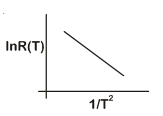# In a photoelectric  effect  experiment the threshold wavelength of light is 380nm.If the wavelength of incident light is 260nm, the maximum kinetic energy of emitted electrons will be: Given  E (in eV)=(a) 4.5eV (b) 15.1eV (c) 3.0eV (d) 1.5eV

## Question ID - 51323 :- In a photoelectric  effect  experiment the threshold wavelength of light is 380nm.If the wavelength of incident light is 260nm, the maximum kinetic energy of emitted electrons will be: Given  E (in eV)=(a) 4.5eV (b) 15.1eV (c) 3.0eV (d) 1.5eV

3537

Wavelength of incident wavesCut off (threshold)wavelength=380nm

Then

K==1237== 1.5eV

Next Question :
 In an experiment, the resistance of a material is plotted as a function of temperature (in some range). As shown in the figure, it is a straight line.One may conclude that: (a) R(T)=(b) R(T)=R0(c) R(T)=R0(d) R(T)=R0